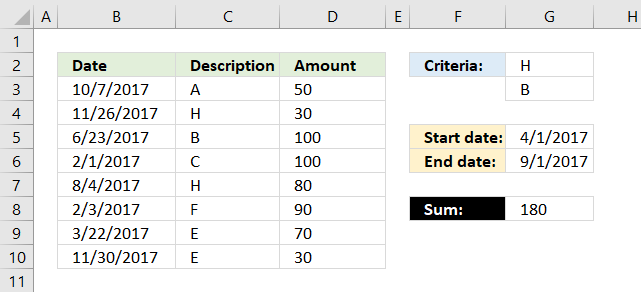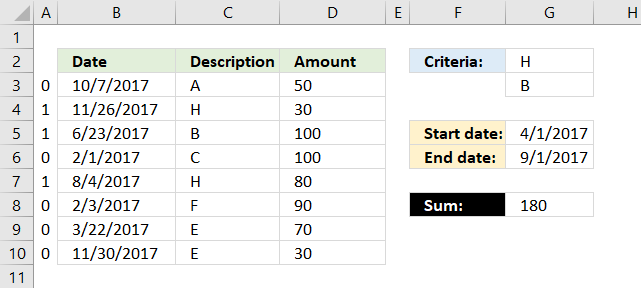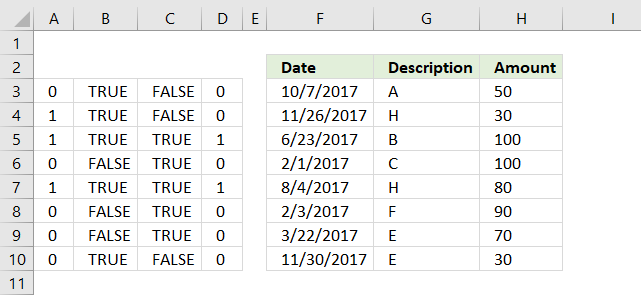Author: Oscar Cronquist Article last updated on December 05, 2018The formula above in cell G8 uses two conditions in cell G2 and G3 and a date range G5:G6 to create a total. The conditions in cell G2 and G3 are matched to the "Description" values in column C and the date range specified in cell G5 and G6 are used to filter values based on the dates in column B.

=SUMPRODUCT(COUNTIF(G2:G3,C3:C10)* (G5<=B3:B10)* (G6>=B3:B10)* D3:D10)

### Explaining formula in cell G8

#### Step 1 - Identify values matching cell G2 and G3

The COUNTIF function allows you to compare multiple values to one column.

COUNTIF(G2:G3, C3:C10)

becomes

COUNTIF({"H";"B"},{"A";"H";"B";"C";"H";"F";"E";"E"})

and returns

{0;1;1;0;1;0;0;0}.

This tells us that the second, third and fifth value in column C matches G2 or G3.

The picture below shows the array in column A.#### Step 2 - Identify values inside date range

To match a date range we need two logical expressions, the first one checks if any of the dates in B3:B10 are larger than or equal to the start date in G5.

(G5<=B3:B10)

returns

{TRUE; TRUE; TRUE; FALSE; TRUE; FALSE; FALSE; TRUE}.

The second logical expression matches dates smaller than or equal to the end date.

(G6>=B3:B10)

returns

{FALSE; FALSE; TRUE; TRUE; TRUE; TRUE; TRUE; FALSE}

#### Step 3 - Apply AND logic to all arraysColumn D shows the result of multiplying all arrays from the logical expressions and the COUNTIF function. This is the same as AND logic for values row-wise.

For example, the first value on row 3 is 0 (zero), the next is TRUE and the last value is FALSE. 0*TRUE*FALSE equals FALSE. All values must be TRUE to return TRUE.

Note, 0 (zero) is the same as FALSE and 1 is TRUE in Excel. Row 5 and 7 in column D return 1.

#### Step 4 - Sum all numbers in array

The SUMPRODUCT function creates a total based on logical expressions.

SUMPRODUCT(COUNTIF(G2:G3,C3:C10)* (G5<=B3:B10)* (G6>=B3:B10)* D3:D10)

becomes

SUMPRODUCT({0;0;1;0;1;0;0;0}* D3:D10)

Lastly, multiply the array with the amounts in column D.

SUMPRODUCT({0;0;1;0;1;0;0;0}* {50; 30; 100; 100; 80; 90; 70; 30})

calculates to

SUMPRODUCT({0;0;100;0;80;0;0;0})

and returns 180 in cell G8.